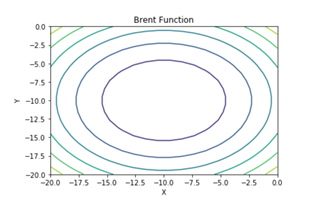top of page
Search

# Brent FunctionMathematical DefinitionInput Domain

The function can be defined on any input range but it is usually evaluated on x ∈ [-20,0] and y ∈ [-20,0].

Global Minima

The function has one global minimum at f(x∗) = e-200 located at x∗=(−10,−10).

Characteristics

• The function is convex.

• The function is defined on 2-dimensional space.

• The function is differentiable.

• The function is non-separable.

Python Implementation

```% Please forward any comments or bug reports in chat
Copyright 2021. INDUSMIC PRIVATE LIMITED.THERE IS NO WARRANTY, EXPRESS OR IMPLIED. WE DO NOT ASSUME ANY LIABILITY FOR THE USE OF THIS PROGRAM. If software is modified to produce derivative works, such modified software should be clearly marked. Additionally, user can redistribute it and/or modify it under the terms of the GNU General Public License. This program is distributed in the hope that it will be useful, but WITHOUT ANY WARRANTY. See the GNU General Public License for more details.
% for any support connect with us on help.indusmic@gmail.com
% Author: SHIVANGI CHANDRA DUBEY

#For   n=2
#brent   accepts the values of 2 MxM dimension matrices X, Y
#it   returns the computation of the matrices in an MxM matrix Z
#the   function is then plotted using (X,Y,Z)
#thus   giving us a contour plot

from mpl_toolkits import mplot3d
%matplotlib inline
import numpy as np
import matplotlib.pyplot as plt
from matplotlib import cm

def   brent(x,y):
return   (x+10)**2+(y+10)**2+np.exp(-x**2-y**2);

x=np.linspace(-20,0,20)
y=np.linspace(-20,0,20)

X,Y=np.meshgrid(x,y)
Z=brent(X,Y)

def   plotFunction(e,a):
fig=plt.figure(figsize=[12,8])
ax=plt.axes(projection='3d')
surf=ax.plot_surface(X,Y,Z,cmap=cm.coolwarm)
ax.view_init(elev=e,azim=a)
ax.set_xlabel('X')
ax.set_ylabel('Y')
ax.set_zlabel('fx')
ax.set_title('Brent Function')
fig.colorbar(surf,   shrink=0.5, aspect=5)
plt.show()
plt.contour(X,Y,Z)
plt.show()

from ipywidgets import interactive
iplot=interactive(plotFunction,
e=(-90,90,5),
a=(-90,90,5))

iplot```

References:

 Survajonic, Sonja & Bingham, Derek, “Virtual Library of Simulation Experiments”, sfu.ca,

https://www.sfu.ca/~ssurjano/optimization.html